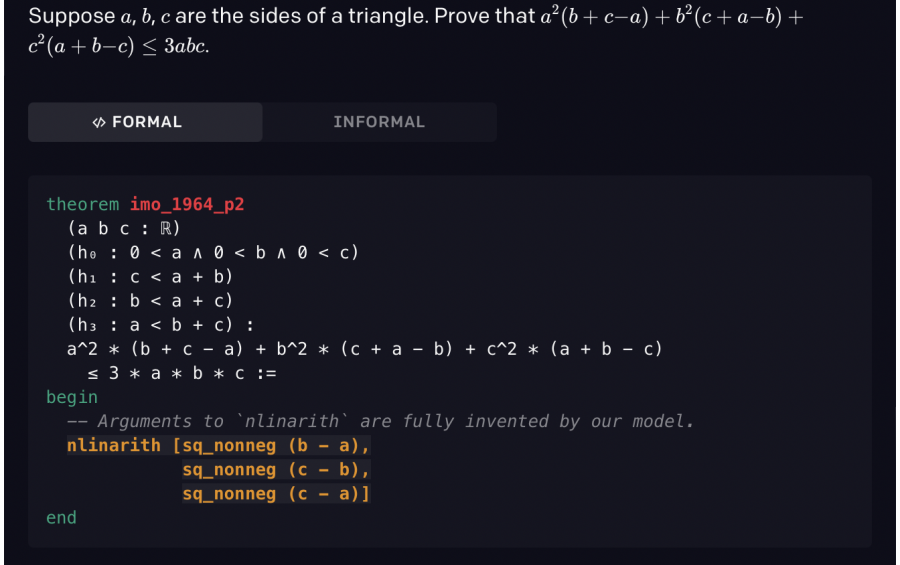• # OpenAI has trained a model to prove theoremsOpen AIn AI presented a neural network proving theorems. The model achieved 41% accuracy on the miniF2F – dataset of school Olympiad problems.

To search for evidence, a language model is used, trained according to the principle of teaching schoolchildren: every time a neural network finds a new proof, it is added to the training dataset, which improves the neural network and allows it to iteratively find solutions to increasingly complex statements.

The best result on the miniF2F benchmark (41.2% vs. 29.3% for the previous state-of-the-art model) was achieved after 8 iterations of training.

OpenAI claims that using reinforcement learning would not allow such a high result due to the infinite space of mathematical actions. Using a language model solves this problem, since language models have the ability to introduce new mathematical constructions that allow solving the problem.

Subscribe
Notify of